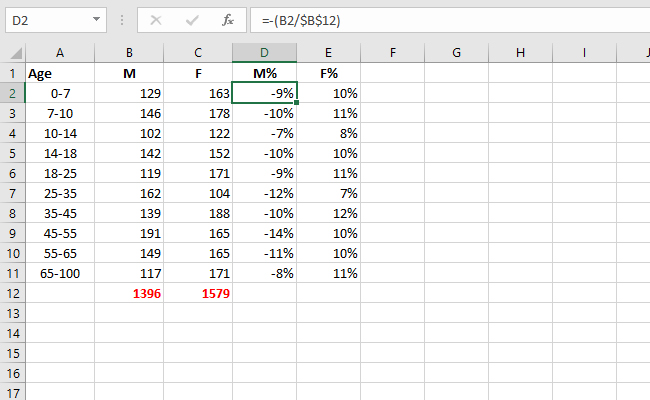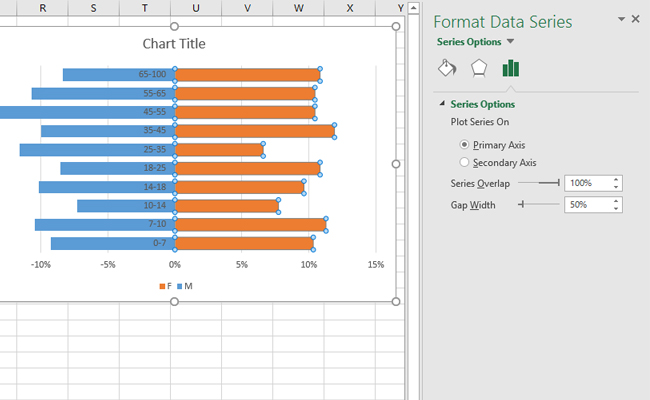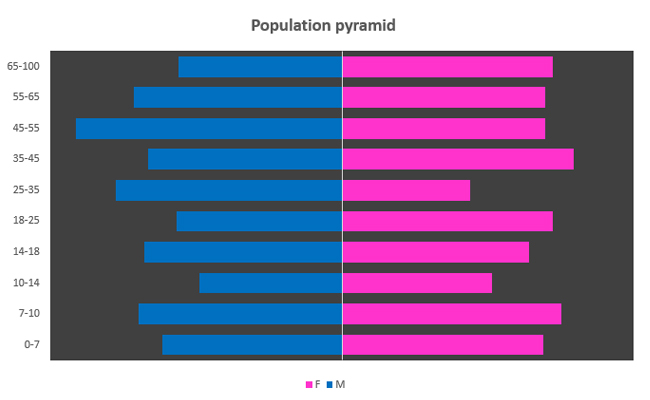# Population pyramid chart

Population pyramid is a subclass of the Bar chart chart that is conenient when we need to show results of the research in which we compare two populations. Most often we compare the percentage representation. These can be answers, in relation to the age, how many survey members responded with the “YES” and how many with “NO”, we can compare the number of men and women which belong to a certain population, etc.

How to create this chart you will see on a simple example. A table was created in which the first column indicates the age range, the second number of men, and the third number of women in the survey. Below the second and third column we created a total of values. In order to create a chart we need to calculate the percentages, which we do by adding two more columns in which the value of each row is divided by the total value. Since we want to show the data about men on the left, we will multiply this column by (-1).Next you need to go to the Insert ribbon, then click on Recommended Charts. In the window that appears first click on the All Charts tab, and then select the option to create a simple Bar Chart. When it appears, we can increase its size according to our preferences.

Since “bars” in Bar Chart are not in line, we have to tune them. We do this by right-clicking one of the series and in the context menu should choose Format Data Series option. The Series Overlap slider should be pulled right to the maximum value of 100%. We can set the Gap Width, which adjusts the width of the bar, to 50%. We got a simple Population pyramid chart!Now we can do some formatting to make it even better: by moving the y label marks to the left, adjusting the background color, coloring the series, deleting the x axes labels etc. As a result we will get an effective chart showing the distribution of the population of men and women.